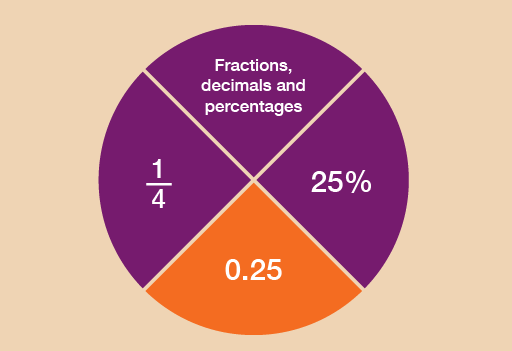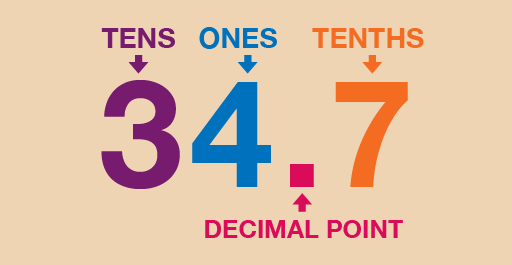Everyday maths 1 (Wales)

Start this free course now. Just create an account and sign in. Enrol and complete the course for a free statement of participation or digital badge if available.

Free course

# 4 DecimalsFigure 20 Looking at decimals

Can you think of any examples of when you might come across decimal numbers in everyday life?

If you’re dealing with money and the decimal point is not placed correctly, then the value will be completely different, for example, £5.55 could be mistaken for £55.50.

Likewise with weights and measures: if the builder in the last activity made a wrong measurement, the whole kitchen extension could be affected.

• the value of a digit in a decimal number
• ways of carrying out calculations with decimal numbers
• approximate answers to calculations involving decimal numbers.

You looked at place value in the section on whole numbers. Now you’ll take a look at decimals.Figure 21 What is a decimal point?

So what is a decimal point?

It separates a number into its whole number and its fractional part. So in the example above, 34 is the whole number, and the seven – or 0.7, as it would be written – is the fractional part.

Each digit in a number has a value that depends on its position in the number. This is its place value:

Whole number part.Fractional part
ThousandsHundredsTensUnits.TenthsHundredthsThousandths
1000s100s10s1s.sss

Look at these examples, where the number after the decimal point is also shown as a fraction:

5.1 = 5 and

67.2 = 67 and

8.01 = 8 and

## Example: Finding values

If you were looking for the place value of each digit in the number 451.963, what would the answer be?

HundredsTensUnits.TenthsHundredthsThousandths
451.963

4 hundreds

5 tens

1 unit

9 tenths ()

6 hundredths ()

3 thousandths ()

## Activity 23: Decimal dilemmas

1. Four children are taken to the funfair. One of the rides, the Wacky Wheel, has the following notice on it:
• For safety reasons, children must be over 0.95 m tall to go on this ride.
• Margaret is 0.85 m tall.
• David is 0.99 m tall.
• Suha is 0.89 m tall.
• Prabha is 0.92 m tall.
• Who is allowed to go on the ride?
1. Six athletes run a race. Their times, in seconds, are as follows:
 Sonia 10.95 Anjali 10.59 Anita 10.91 Aarti 10.99 Sita 10.58 Susie 10.56
• Who gets the gold, silver and bronze medals?
1. In a gymnastics competition, the following points were awarded to four competitors. Who came first, second and third?
 Janak 23.95 Nadia 23.89 Carol 23.98 Tracey 23.88

1. Any child that is more than 0.95 m tall will be allowed on the ride. So to answer the question you need to compare the height of each child with 0.95 m.
TenthsHundredths
Margaret85
David99
Suha89
Prabha92
• Comparing the tenths tells us that only two children may possibly be allowed on the ride: David and Prabha.
• If we go on to compare the hundredths, we see that only David is taller than 0.95 m.
• So only David would be allowed on the Wacky Wheel.
1. You need to compare the tens, units, tenths and hundredths, in that order.
TensUnits.TenthsHundredths
Sonia10.95
Anjali10.59
Anita10.91
Aarti10.99
Sita10.58
Susie10.56
• All of the times have the same number of tens and units, so it is necessary to go on to compare the tenths.
• The three times with the lowest number of tenths are 10.59, (Anjali), 10.58 (Sita) and 10.56 (Susie). If we now go on to compare the hundredths in these three times, we see that the lowest times are (lowest first): 10.56, 10.58 and 10.59.
• So medals go to:
• Susie (10.56 secs): gold
• Sita (10.58 secs): silver
• Anjali (10.59 secs): bronze
1. Again, we need to compare the tens, units, tenths and hundredths, in that order.
TensUnits.TenthsHundredths
Janak23.95
Carol23.98
Tracey23.88
• All the scores have the same number of tens and units. Looking at the tenths, two scores (23.95 and 23.98) have 9 tenths. If you compare the hundredths in these two numbers, you can see that 23.98 is bigger than 23.95.
• To find the third highest number, go back to the other two numbers, 23.89 and 23.88. Comparing the hundredths, you can see that 23.89 is the higher number. So the top three competitors are:
• Carol (23.98)
• Janak (23.95)